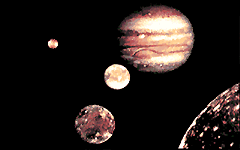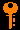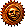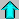# Lecture 6 - Orbital Mechanics II: The Barycenter (9/29/98)Mechanics I --- | --- EnergyChapter 1-5, P1-8 (ZG4)

Moons of Jupiter, Voyager Mosaic (courtesy NASA)
Key Question:## Investigations:

1. Conservation of Angular Momentum
• What is angular momentum?
• What the orbital angular momentum of a binary system?
• Why is angular momentum conserved?
2. The Center of Mass
• What is meant by center of mass of a system of particles?
• What is an inertial frame?
• What is the barycenter?
• What is the reduced mass?
3. Kepler's Laws Revisited
• What is at the focus of an orbit?
• Why are orbits conic sections?
• What is the basis of Kepler's Second Law?
• How does Kepler's Third Law come about?

## The Center of Mass:

Because of Newton's third Law of reaction, if the Earth is made to orbit because of the gravitational force between the Earth and Sun, then the Sun must also orbit by the equal and opposite force. Because the forces are equal, then the accelerations are inversely proportional to the masses, and we would expect the two bodies to each orbit a point on the line between them, but closer to the more massive body (the Sun).

We will consider circular orbits as usual.

To see this, equate the centripetal forces on two masses m_1 and m_2 orbiting at distances r_1 and r_2 respectively from the orbital center:

m1 v12 / r1 = m2 v22 / r2

To remain on opposite sides of the orbital center, which they must do to for force symmetry reasons, then the periods of the orbits must be the same:

P = 2 Pi r1 / v1 = 2 Pi r2 / v2

and thus

r1 / v1 = r2 / v2.

Combining with the previous equation for v_1^2 / v_2^2, we get

m1 r1 = m2 r2

which is the equation for the center of mass of two bodies. The relative distances from the center of mass are inversely proportional to the respective masses.

Because the mass of the Sun (2 × 10^30 kg) is so much greater than that of the Earth (6 × 10^24 kg), the center of mass of the Earth-Sun orbit lies very close to the Sun:

sun / rearth = mearth / msun = (6 × 1024 kg) / (3 × 1030 kg) = 3 × 10-6

Since the sum of the distances r_s + r_e = 1 AU = 1.5 × 10^8 km, then r_s = 450 km, which is still very close to the center of the Sun! Q: What is the distance of the center-of-mass of the Earth-Moon system from the center of the Earth?

For two orbiting bodies, the center of mass is called the barycenter. The Sun and Earth mutually revolve around the Sun-Earth barycenter.

## Kepler's Third Law (revisited):

Because two bodies mutually revolve about their barycenter, Newton knew he had to modify Kepler's third law to take this into account. If we equate the centripetal force about the barycenter with the gravitational force between the bodies, then we find

G m1 m2 / a2 = m1 v12 / r1
= m1 r1 ( v1 / r1 )2
= m1 r1 ( 2 pi / P )2

where

a = r1 + r2

is the semimajor axis of the orbit. The equation for the center of mass tells us

m1 r1 = m2 r2 = m2 ( a - r1 )

so

r1 = a m2 / (m1 + m2)

and upon substitution

G m1 m2 / a2 = ( 2 pi / P )2 m1 m2 a / (m1 + m2)

and so

G (m1 + m2) P2 = 4 pi2 a3

which is Kepler's 3rd law but now with both masses included. If we define the total mass of the system to be

M = m1 + m2

then we have simply

G M P2 = 4 pi2 a3.

Note that this is valid for elliptical as well as circular orbits.

We can get an easier equation by using the Earth's orbit to scale things:

(M / Msun ) (P / 1 yr)2 = (a / 1 AU)3

Remember to use masses in units of the Sun's mass, periods in units of years, and distances in units of AU, and you can simply write:

M P2 = a3

Do not measure masses in kilograms for this equation!Prev Lecture ---Next Lecture ---Astr11 Index ---Astr11 Home

smyers@nrao.edu Steven T. Myers